# Image Processing with Wavelets¶

Important: Please read the installation page for details about how to install the toolboxes. $\newcommand{\dotp}{\langle #1, #2 \rangle}$ $\newcommand{\enscond}{\lbrace #1, #2 \rbrace}$ $\newcommand{\pd}{ \frac{ \partial #1}{\partial #2} }$ $\newcommand{\umin}{\underset{#1}{\min}\;}$ $\newcommand{\umax}{\underset{#1}{\max}\;}$ $\newcommand{\umin}{\underset{#1}{\min}\;}$ $\newcommand{\uargmin}{\underset{#1}{argmin}\;}$ $\newcommand{\norm}{\|#1\|}$ $\newcommand{\abs}{\left|#1\right|}$ $\newcommand{\choice}{ \left\{ \begin{array}{l} #1 \end{array} \right. }$ $\newcommand{\pa}{\left(#1\right)}$ $\newcommand{\diag}{{diag}\left( #1 \right)}$ $\newcommand{\qandq}{\quad\text{and}\quad}$ $\newcommand{\qwhereq}{\quad\text{where}\quad}$ $\newcommand{\qifq}{ \quad \text{if} \quad }$ $\newcommand{\qarrq}{ \quad \Longrightarrow \quad }$ $\newcommand{\ZZ}{\mathbb{Z}}$ $\newcommand{\CC}{\mathbb{C}}$ $\newcommand{\RR}{\mathbb{R}}$ $\newcommand{\EE}{\mathbb{E}}$ $\newcommand{\Zz}{\mathcal{Z}}$ $\newcommand{\Ww}{\mathcal{W}}$ $\newcommand{\Vv}{\mathcal{V}}$ $\newcommand{\Nn}{\mathcal{N}}$ $\newcommand{\NN}{\mathcal{N}}$ $\newcommand{\Hh}{\mathcal{H}}$ $\newcommand{\Bb}{\mathcal{B}}$ $\newcommand{\Ee}{\mathcal{E}}$ $\newcommand{\Cc}{\mathcal{C}}$ $\newcommand{\Gg}{\mathcal{G}}$ $\newcommand{\Ss}{\mathcal{S}}$ $\newcommand{\Pp}{\mathcal{P}}$ $\newcommand{\Ff}{\mathcal{F}}$ $\newcommand{\Xx}{\mathcal{X}}$ $\newcommand{\Mm}{\mathcal{M}}$ $\newcommand{\Ii}{\mathcal{I}}$ $\newcommand{\Dd}{\mathcal{D}}$ $\newcommand{\Ll}{\mathcal{L}}$ $\newcommand{\Tt}{\mathcal{T}}$ $\newcommand{\si}{\sigma}$ $\newcommand{\al}{\alpha}$ $\newcommand{\la}{\lambda}$ $\newcommand{\ga}{\gamma}$ $\newcommand{\Ga}{\Gamma}$ $\newcommand{\La}{\Lambda}$ $\newcommand{\si}{\sigma}$ $\newcommand{\Si}{\Sigma}$ $\newcommand{\be}{\beta}$ $\newcommand{\de}{\delta}$ $\newcommand{\De}{\Delta}$ $\newcommand{\phi}{\varphi}$ $\newcommand{\th}{\theta}$ $\newcommand{\om}{\omega}$ $\newcommand{\Om}{\Omega}$

This numerical tour overviews the use of wavelets for image approximation and denoising.

In :
addpath('toolbox_signal')


## Wavelet Approximation¶

Image approximation is obtained by thresholding wavelets coefficients.

First we load an image $f \in \mathbb{R}^N$ of $N = n_0 \times n_0$ pixels.

In :
name = 'cortex';
n0 = 512;
f = rescale( sum(f,3) );


Display it.

In :
clf;
imageplot(f);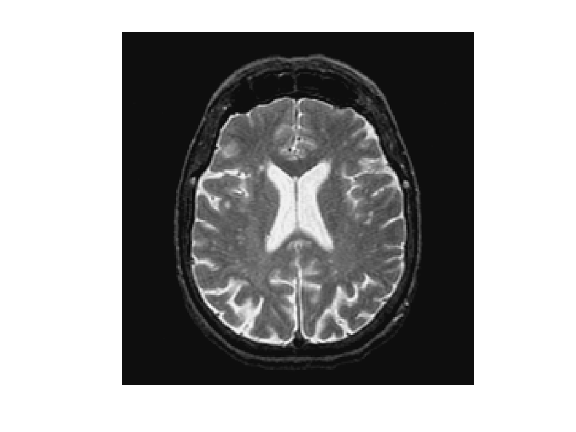An orthogonal wavelet basis $\mathcal{B} = \{ \psi_{j,n}^k \}_{j,n}$ of $\mathbb{R}^N$ is composed of multiscale atoms parameterized by their scale $2^j$, position $2^j n \in [0,1]^2$ and orentation $k \in \{H,V,D\}$.

A forward wavelet transform computes the set of inner products $$\Psi f = \{ \langle f,\psi_{j,n}^k\rangle \} \in \mathbb{R}^N$$ where the wavelet atoms are defined as $$\psi_{j,n}^k(x) = \psi^k\left( \frac{x - 2^j n}{2^j} \right).$$

Set the minimum scale for the transform.

In :
Jmin = 0;


A short-cut for the wavelet transform $\Psi$:

In :
Psi = @(f)perform_wavelet_transf(f,Jmin,+1);


A short-cut for the inverse wavelet transform $\Psi^{-1} = \Psi^*$:

In :
PsiS = @(fw)perform_wavelet_transf(fw,Jmin,-1);


Perform the wavelet transform to compute $\Psi f$.

In :
fW = Psi(f);


Display the transformed coefficients.

In :
clf;
plot_wavelet(fW);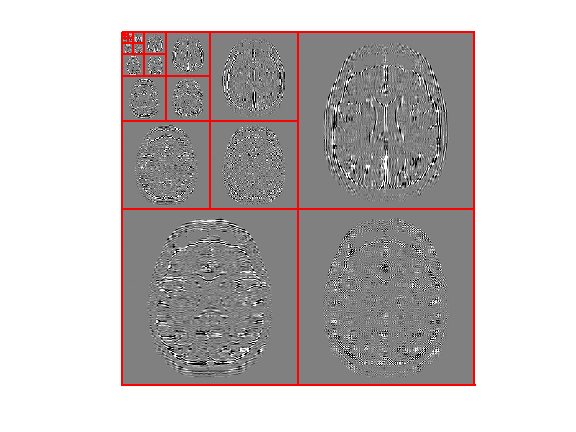To perform non-linear image approximation, one remove the small amplitude coefficients. This is performed using a hard thresholding $$H_T(f,\mathcal{B}) = \Psi^{-1} \circ H_T \circ \Psi (f) = \sum_{|\langle f,\psi_{j,n}^k\rangle| > T} \langle f,\psi_{j,n}^k\rangle \psi_{j,n}^k.$$

$T$ should be adapted to ensure a given number $M$ of non-zero coefficients, and then $f_M = H_T(f,\mathcal{B})$ is the best $M$ terms approximation of $f$ in $\mathcal{B}$.

Select a threshold.

In :
T = .5;


Shortcut for the thresholding operator $H_T$.

In :
Thresh = @(fW,T)fW .* (abs(fW)>T);


Perform hard thresholding of the coefficients.

In :
fWT = Thresh(fW,T);


Exercise 1

Compute the ratio $M/N$ of non-zero coefficients.

In :
exo1()

Ratio of non-zero coefficients : M/N = 0.00481.

In :
%% Insert your code here.


Display the thresholded coefficients.

In :
clf;
subplot(1,2,1);
plot_wavelet(fW);
title('Original coefficients');
subplot(1,2,2);
plot_wavelet(fWT);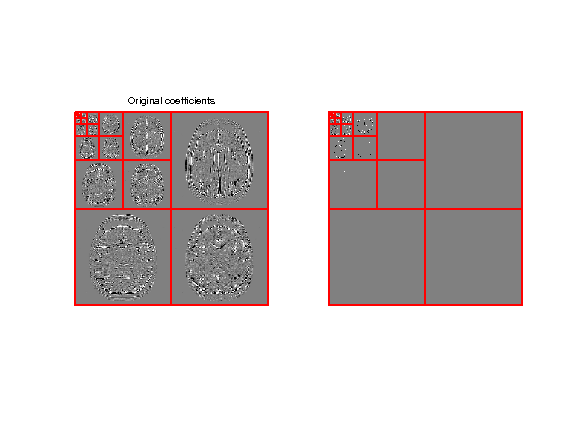Perform reconstruction using the inverse wavelet transform $\Psi^*$.

In :
f1 = PsiS(fWT);


Display approximation.

In :
clf;
imageplot(f, 'Image', 1,2,1);
imageplot(clamp(f1), strcat(['Approximation, SNR=' num2str(snr(f,f1),3) 'dB']), 1,2,2);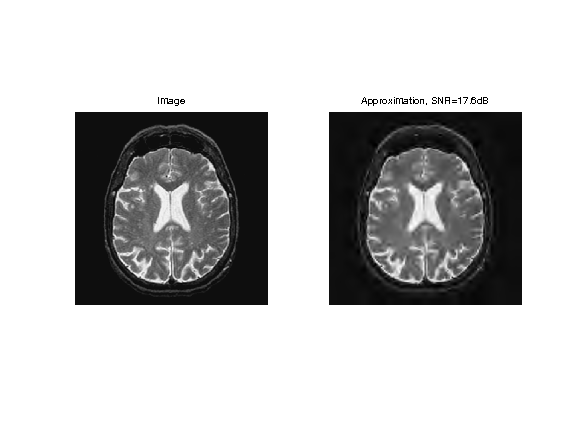Number of coefficients for the approximation.

In :
M = n0^2/16;


Exercise 2

Compute a threshold $T$ to keep only $M$ coefficients.

In :
exo2()

In :
%% Insert your code here.


Perform hard thresholding.

In :
fWT = Thresh(fW,T);


Check the number of non-zero coefficients in |fWT|.

In :
disp(strcat(['      M=' num2str(M)]));
disp(strcat(['|fWT|_0=' num2str(sum(fWT(:)~=0))]));

      M=16384
|fWT|_0=16384


Exercise 3

Compute an approximation with an decreasing number of coefficients.

In :
exo3()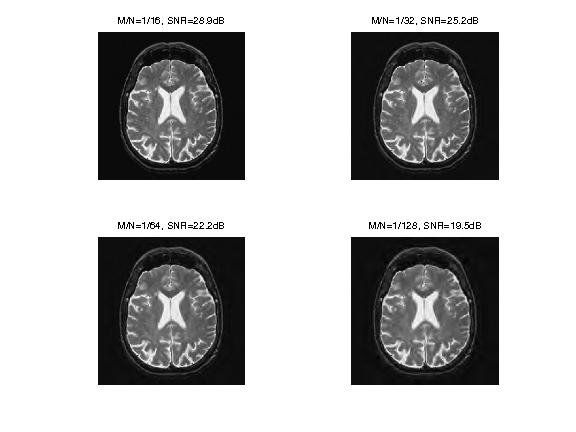In :
%% Insert your code here.


## Orthognal Wavelet Denoising¶

Image denoising is obtained by thresholding noisy wavelets coefficielts.

Here we consider a simple setting where we intentionnaly add some noise $w$ to a clean image $f$ to obtain $y = f + w$.

A denoiser computes an estimate $\tilde f$ of $f$ from the observations $y$ alone. In the mathematical model, since $y$ is a random variable depending on $w$, so is $\tilde f$. A mathematical evaluation of the efficiency of the denoiser is the average risk $E_w( \|f-\tilde f\|^2 )$.

Here we consider a single realization of the noise, so we replace the risk by the oracle error $\|f-\tilde f\|^2$. This allows us to bench the efficiency of the denoising methods by comparing the result to $f$. But you have to keep in mind that for real application, one does not have access to $f$.

We consider a Gaussian white noise $w$ of variance $\sigma^2$.

In :
sigma = .1;


Generate a noisy image.

In :
y = f + randn(n0,n0)*sigma;


Display.

In :
clf;
imageplot(f, 'Clean image', 1,2,1);
imageplot(clamp(y), ['Noisy image, SNR=' num2str(snr(f,y),3) 'dB'], 1,2,2);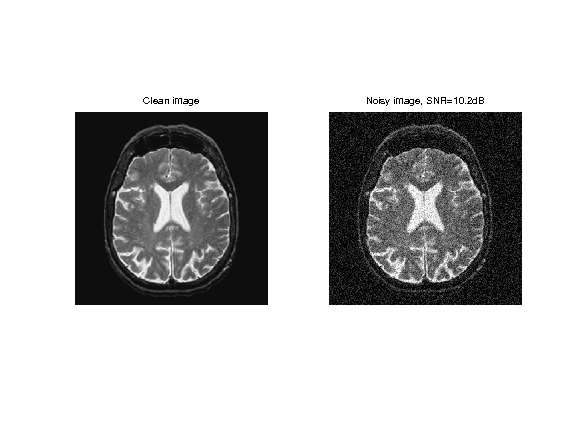A denoising is obtained by thresholding the wavelet coefficients $$\tilde f = H_T(f,\mathcal{B}).$$

The asymptotically optimal threshold of Donoho and Johnstone is $T = \sqrt{2 \log(N)} \sigma$. In practice, one observes that much better result are obtained using $T \approx 3 \sigma$.

Compute the noisy wavelet coefficients.

In :
fW = Psi(y);


Compute the threshold value using the $3\sigma$ heuristic.

In :
T = 3*sigma;


Perform hard thresholding.

In :
fWT = Thresh(fW,T);


Display the thresholded coefficients.

In :
clf;
subplot(1,2,1);
plot_wavelet(fW);
title('Original coefficients');
subplot(1,2,2);
plot_wavelet(fWT);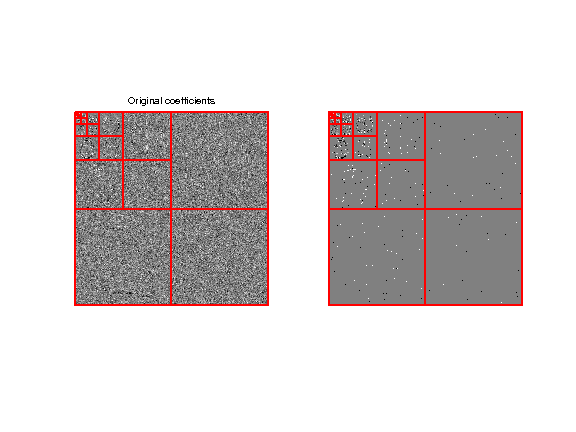Perform reconstruction.

In :
f1 = PsiS(fWT);


Display denoising.

In :
clf;
imageplot(clamp(y), 'Noisy image', 1,2,1);
imageplot(clamp(f1), strcat(['Denoising, SNR=' num2str(snr(f,f1),3) 'dB']), 1,2,2);